Published

FATBB Autonomous Vehicle

In this project, our team built a lane following, autonomous RC car with the beagle bone black using the OpenCV library.

IntermediateShowcase (no instructions)20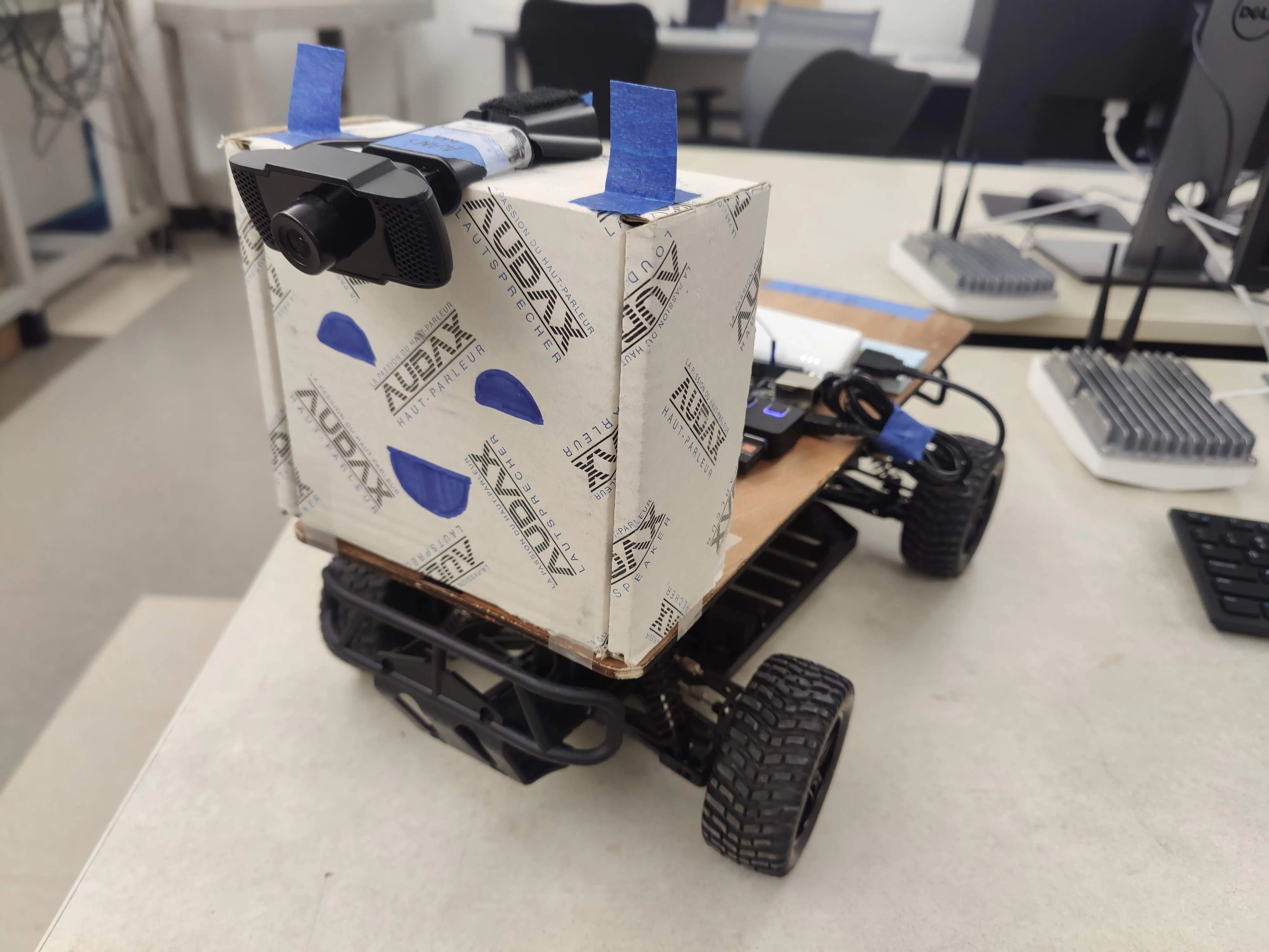Things used in this project

Hardware components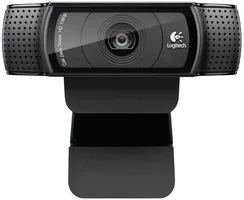Webcam, Logitech® HD Pro
×1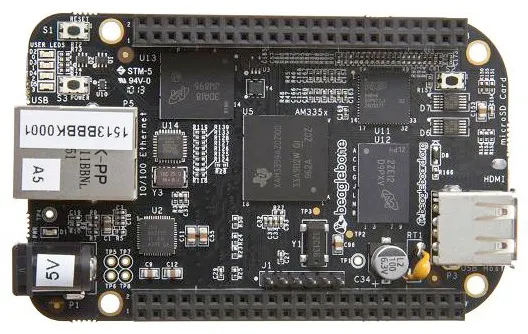BeagleBoard.org BeagleBone Black
×1USB Expansion HUB, USB 2.0 Powered
×1
×1

Software apps and online servicesOpenCV

Schematics

img_20211212_143817991_Rqe35VLghZ.jpg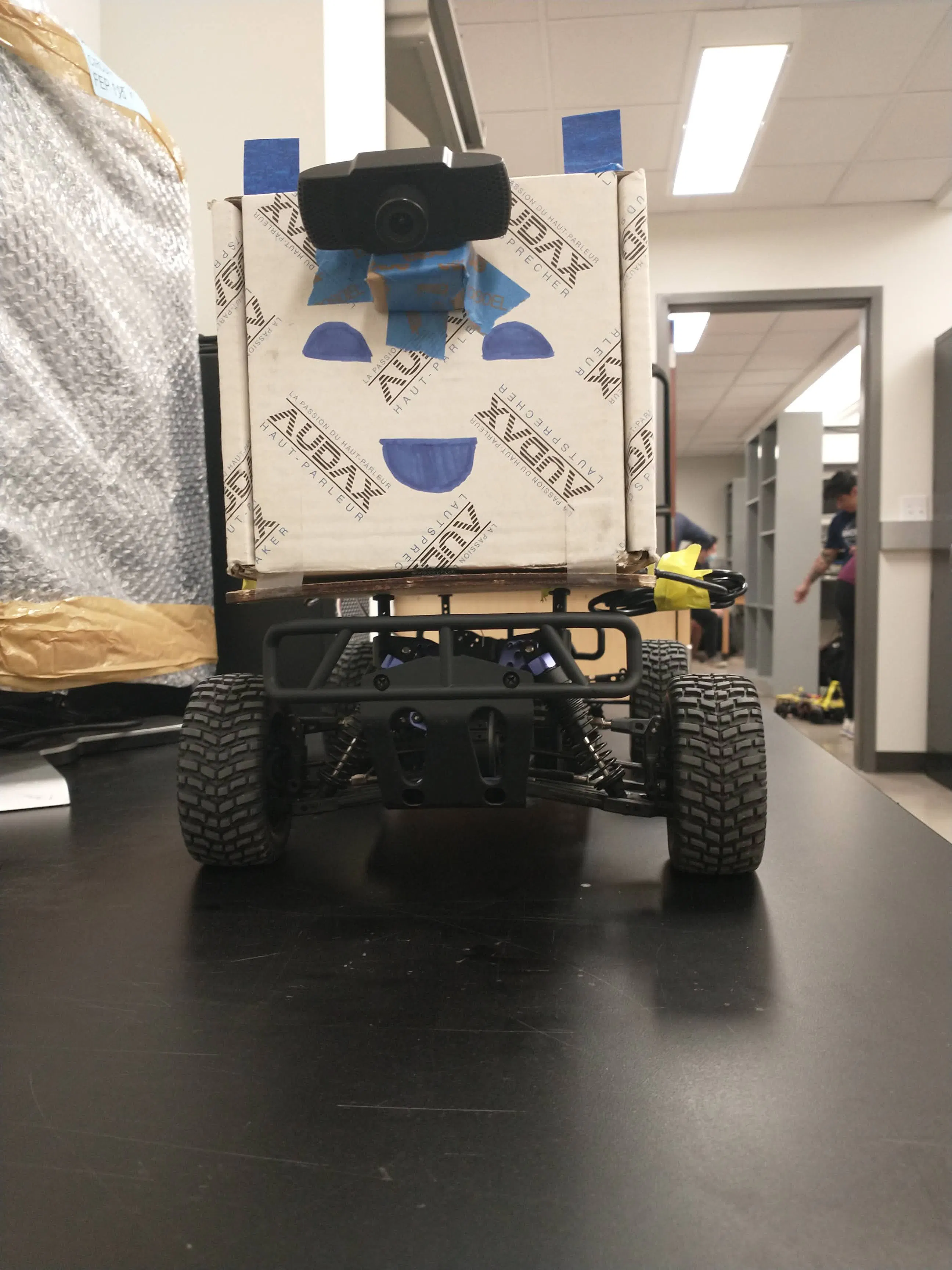img_20211212_143813477_2xP5MV4POi.jpg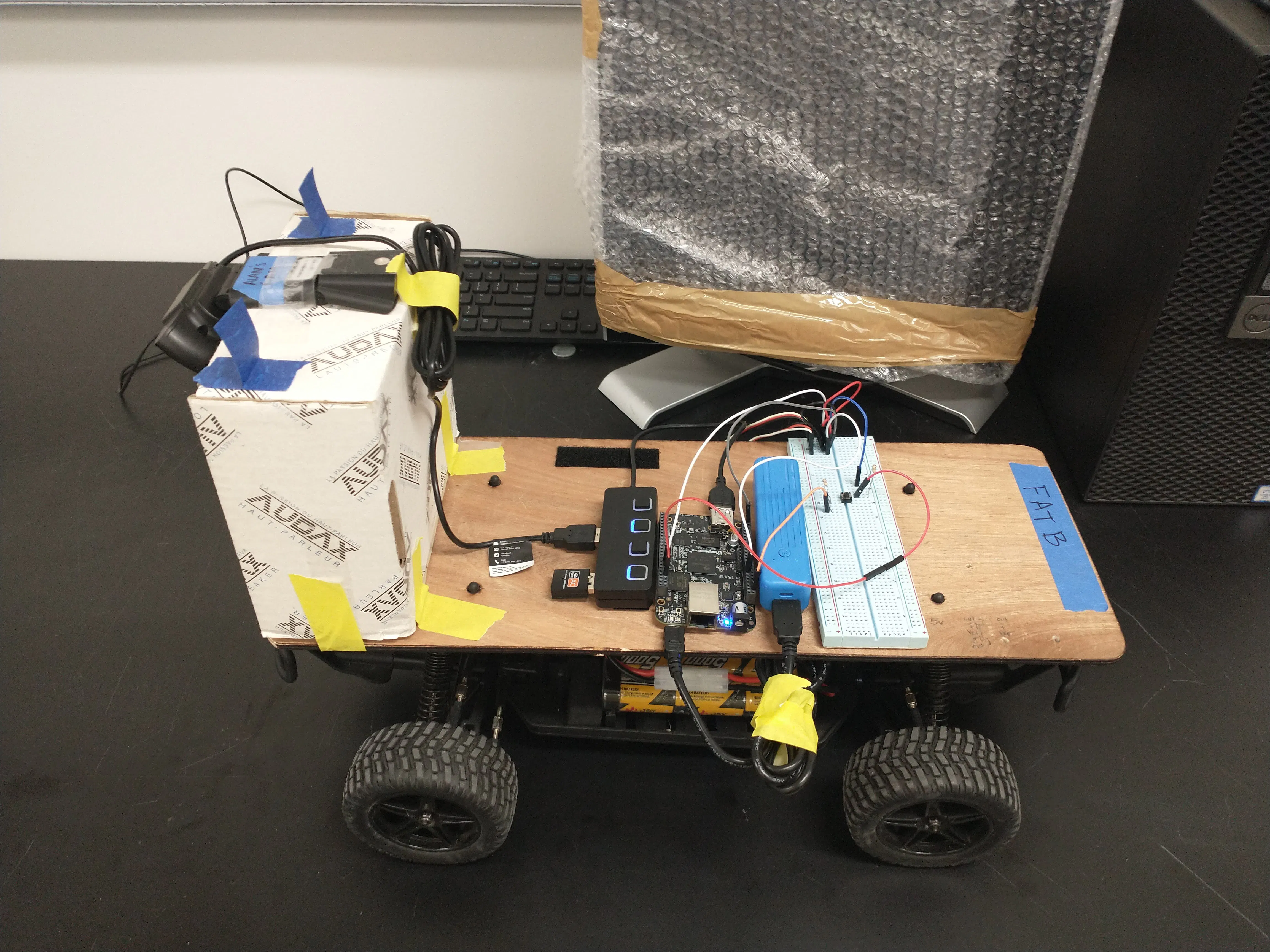img_20211212_143810202_v2qLhUg1Mx.jpg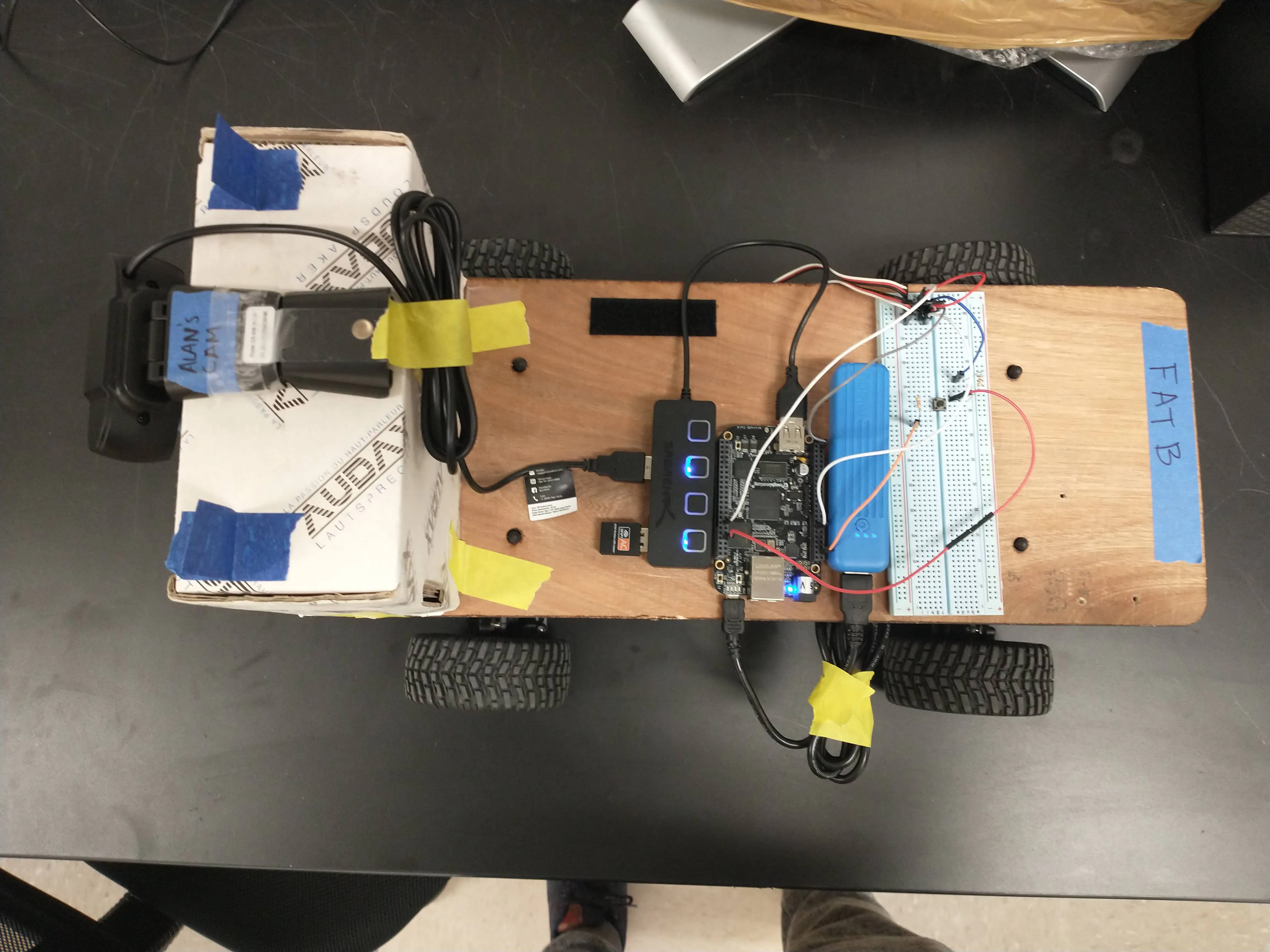Code

SelfDrive.py

Python
This is the code to run the entire car
#A Huge thank you to: User raja_961, Autonomous Lane-Keeping Car Using Raspberry Pi and OpenCV. Instructables.
#Code was modified from raja_961's.

import cv2
import numpy as np
import math
import sys
import time

#throttle and steering and button interrupt setup
throttlePin = "P9_14" # Physical pin P9_14
steeringPin = "P8_13" # Physical Pin P8_13
button = "P8_7"

#frame count
i = 0

#number of stops seen
signs_seen = 0

#PD Coefficients and speed set up
kp = 2.5
kd = 0.4
speed = 7.95

#GPIO set up for interrupt
GPIO.setup(button, GPIO.IN)

# Steering init
PWM.start(steeringPin, 7.5 ,50,0)

# Throttle init
PWM.start(throttlePin, 7.5, 50, 0)

def detect_edges(frame):
# filter for blue lane lines
hsv = cv2.cvtColor(frame, cv2.COLOR_BGR2HSV)
lower_blue = np.array([90, 120, 0], dtype = "uint8")
upper_blue = np.array([150, 255, 255], dtype="uint8")

# detect edges

return edges

def region_of_interest(edges):
height, width = edges.shape

# only focus lower half of the screen
polygon = np.array([[
(0, height),
(0,  height/2),
(width , height/2),
(width , height),
]], np.int32)

#cv2.imshow("roi",cropped_edges)

return cropped_edges

def detect_line_segments(cropped_edges):
rho = 1
theta = np.pi / 180
min_threshold = 10

line_segments = cv2.HoughLinesP(cropped_edges, rho, theta, min_threshold,
np.array([]), minLineLength=5, maxLineGap=150)

return line_segments

def average_slope_intercept(frame, line_segments):
lane_lines = []

if line_segments is None:
print("no line segments detected")
return lane_lines

height, width,_ = frame.shape
left_fit = []
right_fit = []

boundary = 1/3
left_region_boundary = width * (1 - boundary)
right_region_boundary = width * boundary

for line_segment in line_segments:
for x1, y1, x2, y2 in line_segment:
if x1 == x2:
print("skipping vertical lines (slope = infinity")
continue

fit = np.polyfit((x1, x2), (y1, y2), 1)
slope = (y2 - y1) / (x2 - x1)
intercept = y1 - (slope * x1)

if slope < 0:
if x1 < left_region_boundary and x2 < left_region_boundary:
left_fit.append((slope, intercept))
else:
if x1 > right_region_boundary and x2 > right_region_boundary:
right_fit.append((slope, intercept))

left_fit_average = np.average(left_fit, axis=0)
if len(left_fit) > 0:
lane_lines.append(make_points(frame, left_fit_average))

right_fit_average = np.average(right_fit, axis=0)
if len(right_fit) > 0:
lane_lines.append(make_points(frame, right_fit_average))

return lane_lines

def make_points(frame, line):
height, width, _ = frame.shape

slope, intercept = line

y1 = height  # bottom of the frame
y2 = int(y1 / 2)  # make points from middle of the frame down

if slope == 0:
slope = 0.1

x1 = int((y1 - intercept) / slope)
x2 = int((y2 - intercept) / slope)

return [[x1, y1, x2, y2]]

def display_lines(frame, lines, line_color=(0, 255, 0), line_width=6):
line_image = np.zeros_like(frame)

if lines is not None:
for line in lines:
for x1, y1, x2, y2 in line:
cv2.line(line_image, (x1, y1), (x2, y2), line_color, line_width)

line_image = cv2.addWeighted(frame, 0.8, line_image, 1, 1)

return line_image

def display_heading_line(frame, steering_angle, line_color=(0, 0, 255), line_width=5 ):
height, width, _ = frame.shape

steering_angle_radian = steering_angle / 180.0 * math.pi

x1 = int(width / 2)
y1 = height
x2 = int(x1 - height / 2 / math.tan(steering_angle_radian))
y2 = int(height / 2)

cv2.line(heading_image, (x1, y1), (x2, y2), line_color, line_width)

def get_steering_angle(frame, lane_lines):

height,width,_ = frame.shape

if len(lane_lines) == 2:
_, _, left_x2, _ = lane_lines
_, _, right_x2, _ = lane_lines
mid = int(width / 2)
x_offset = (left_x2 + right_x2) / 2 - mid
y_offset = int(height / 2)

elif len(lane_lines) == 1:
x1, _, x2, _ = lane_lines
x_offset = x2 - x1
y_offset = int(height / 2)

elif len(lane_lines) == 0:
x_offset = 0
y_offset = int(height / 2)

angle_to_mid_deg = int(angle_to_mid_radian * 180.0 / math.pi)
steering_angle = angle_to_mid_deg + 90

return steering_angle

video = cv2.VideoCapture(0)

video.set(cv2.CAP_PROP_FRAME_WIDTH,320)
video.set(cv2.CAP_PROP_FRAME_HEIGHT,240)
#video.set(cv2.CAP_PROP_BUFFERSIZE, 3)
time.sleep(1)

lastTime = 0
lastError = 0

while True:
i = i+1
frame = cv2.resize(frame, (100,75),interpolation=cv2.INTER_AREA)
#Decrease cam resolution for better performance

edges = detect_edges(frame)
roi = region_of_interest(edges)
line_segments = detect_line_segments(roi)
lane_lines = average_slope_intercept(frame,line_segments)
lane_lines_image = display_lines(frame,lane_lines)
steering_angle = get_steering_angle(frame, lane_lines)

#Button Interrupt
if GPIO.input(button):
PWM.set_duty_cycle(throttlePin, 7.5)
print("button pressed")
break

now = time.time()
dt = now - lastTime

#Get steering angle
deviation = steering_angle - 90
error = abs(deviation)
#default steer is 7.5 (straight)
set_steer = 7.5

#START OF STOP SIGN CODE
if i % 10 == 0:
#check every 10 frames for stop sign
hsv_red = cv2.cvtColor(frame, cv2.COLOR_BGR2HSV)

# define range of red color in HSV
lower_red = np.array([160,50,50])
upper_red = np.array([180,255,255])

#Threshold the HSV image to get only red colors, count pixels remaining

#if pixel count is significant and a stop sign has not recently been seen, stop
if(countRed > 500 and i > 20):
PWM.set_duty_cycle(throttlePin, 7.5)
time.sleep(2)
#if this is the second sign, stop forever
if(signs_seen == 1):
print("all done")
while(1):
#Just hang out here forever
print(":D")
signs_seen = 1
#increase speed, P coefficient for turn and restart
speed = speed + 0.02
kp = 5
PWM.set_duty_cycle(throttlePin, speed)
i = 0 #reset frame count

#END OF STOP SIGN CODE

if deviation < 3 and deviation > -3:
deviation = 0
error = 0
#10 degree error range

#PD logic
derivative = kd * (error - lastError) / dt
proportional = kp * error
PD = (int(derivative + proportional)) / 60

#set correct steering angle
if deviation >= 3:
set_steer = set_steer - PD
elif deviation <= -3:
set_steer = set_steer + PD

#max out at full left/full right turns
if set_steer > 9:
set_steer = 9
if set_steer < 6:
set_steer = 6

#turn and go
PWM.set_duty_cycle(steeringPin, set_steer)
PWM.set_duty_cycle(throttlePin, speed)

lastError = error
lastTime = time.time()

key = cv2.waitKey(1)
if key == 27:
break

#Clean up for end of code
video.release()
cv2.destroyAllWindows()
PWM.set_duty_cycle(steeringPin, 7.5)
PWM.set_duty_cycle(throttlePin, 7.5)
PWM.stop(throttlePin)
PWM.stop(steeringPin)

Credits

Alan Lopez

1 project • 0 followers

BenZaltsman

1 project • 1 follower
I love Ray Simar AND Joe Young

Freddy Angarita - Cuesta

1 project • 0 followers